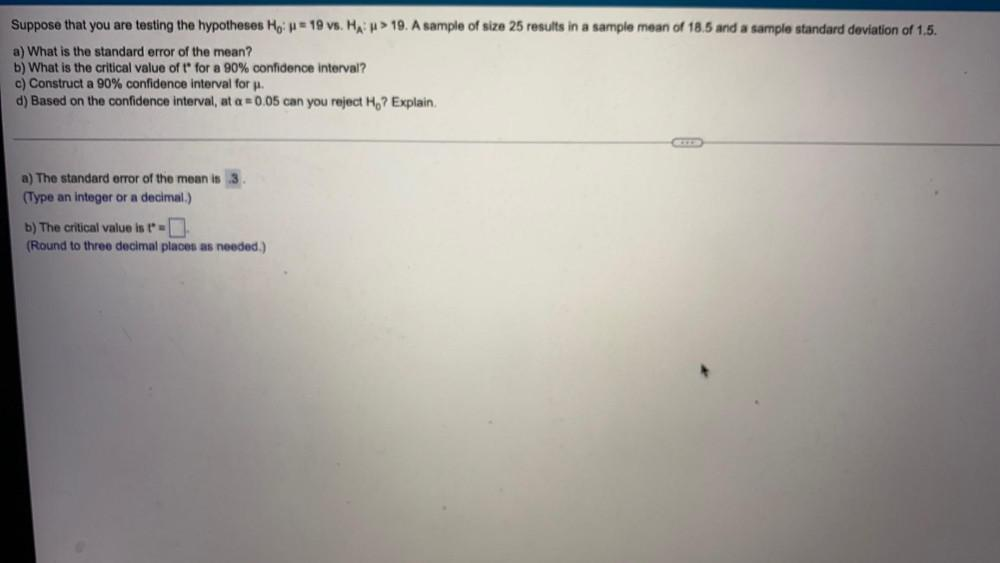Question:

# Suppose that you are testing the hypotheses Ho: = 19 vs. HA: > 19. A sample of size 25 results in a sample mean of 18.5 and a sample standard deviation of 1.5. a) What is the standard error of the meSuppose that you are testing the hypotheses Ho: = 19 vs. HA: > 19. A sample of size 25 results in a sample mean of 18.5 and a sample standard deviation of 1.5. a) What is the standard error of the mean? b) What is the critical value of t for a 90% confidence interval? c) Construct a 90% confidence interval for μ. d) Based on the confidence interval, at a = 0.05 can you reject Ho? Explain. a) The standard error of the mean is 3 b) The critical value is t